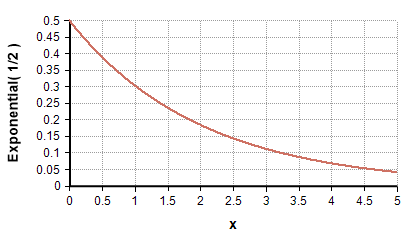# Exponential distribution

The Exponential distribution is a continuous positive-only, univariate distribution that describes the distribution of times between successive independent events in a Poisson process with an average rate of «r» events per unit time. The rate «r» is the reciprocal of the mean of the Poisson distribution—the average number of events per unit time. Its standard deviation is also 1/r.

A model with exponentially distributed times between events is said to be Markov, implying that knowledge about when the next event occurs does not depend on the system's history or how much time has elapsed since the previous event. More general distributions such as the gamma or Weibull do not exhibit this property.## Functions

### Exponential( rate, over )

The distribution function. Use this function to describe a quantity that has an exponential distribution.

To describe independent variables over one or more indexes, list the indexes in the «over» parameter. For example,

Exponential( r, over: Region, Technology )

### Dens_Exponential( x, rate )

To use this, you need to add the Distribution Densities Library to your model.

The analytic probability density at «x», given by

$\displaystyle{ p(x) = r e^{-rx} }$

### CumExponential(x, rate )

The analytic cumulative probability at «x», which returns the probability that an exponentially-distributed quantity with rate «rate» is less than or equal to «x». This is equal to

$\displaystyle{ F(x) = 1 - e^{-r x} }$

### CumExponentialInv(p, rate )

The inverse cumulative probability function (quantile function), given the value x where the probability of the value being less than or equal to x is «p». This is equal to

$\displaystyle{ F^{-1}(p) = {1\over r} \ln(1-p) }$

## Density functions

The probability density of Exponential(r) for $\displaystyle{ x \geq 0 }$ is given by

p(x) = r*Exp(-r*x)

The cumulative density function (CDF) is given by

F(x) = 1 - Exp(-r*x)

## Statistics

The theoretical statistics (i.e., in the absence of sampling error) for the exponential with a «rate» r are as follows.

## Parameter Estimation

Suppose you have sampled historic data in X, indexed by I, with each measurement being a positive real number, and you want to estimate the parameter for the best-fit exponential distribution. The parameter can be estimated using:

1/Mean(X, I)